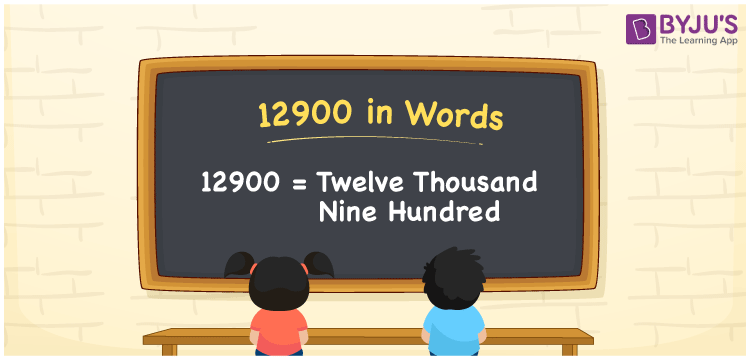# 12900 in Words

We can write 12900 in words is Twelve thousand nine hundred. We can easily convert the number 12900 into words using a place value chart. Suppose you have seen the cardinal number 12900 on a paper; you may pronounce it Twelve thousand nine hundred. In this article, you will understand how to write 12900 in word form.

 12900 in words Twelve thousand nine hundred Twelve thousand nine hundred in numerical form 12900

## 12900 in English Words

Generally, we use English alphabets to write numbers in words and therefore, we can write 12900 in English words as “Twelve thousand nine hundred”.## How to Write 12900 in Words?

We can see that 12900 has five digits, so we need to make a five-column place value chart as shown below.

 Ten thousands Thousands Hundreds Tens Ones 1 2 9 0 0

From the above table, we can expand the digits as per the place values. This can be done as follows.

So, ones = 0, tens = 0, hundreds = 9, thousands = 2, ten thousands = 1

1 × Ten thousand + 2 × Thousand + 9 × Hundred + 0 × Ten + 0 × One

= 1 × 10000 + 2 × 1000 + 9 × 100 + 0 × 10 + 0 × 1

= 10000 + 2000 + 900

= Ten Thousand + Two thousand + Nine hundred

= Twelve thousand + Nine hundred {since ten + two = twelve}

= Twelve thousand nine hundred

12900 is a natural number that is the successor of 12899 and predecessor of 12901.

12900 in words – Twelve thousand nine hundred

Is 12900 an odd number? – No

Is 12900 an even number? – Yes

Is 12900 a prime number? – No

Is 12900 a composite number? – Yes

Is 12900 a perfect square number? – No

Is 12900 a perfect cube number? – No

## Frequently Asked Questions on 12900 in Words

Q1

### How do you write 12900 in words?

The number 12900 is written in words as Twelve thousand nine hundred.
Q2

### How to write Rs. 12900 on a cheque?

On a cheque, Rs. 12900 can be written as “Twelve thousand nine hundred rupees only”.
Q3

### What number is Twelve thousand nine hundred in words?

The number 12900 is Twelve thousand nine hundred in words, which means 12900 = Twelve thousand nine hundred.
Test your Knowledge on 12900 in Words